20.1
19.2
19.1
18.2
18.1
17.2

# Creating Window Calculations

The Dashboard Designer allows you to add a window calculation for numeric measures. To do this, invoke the data item menu and select the required calculation type.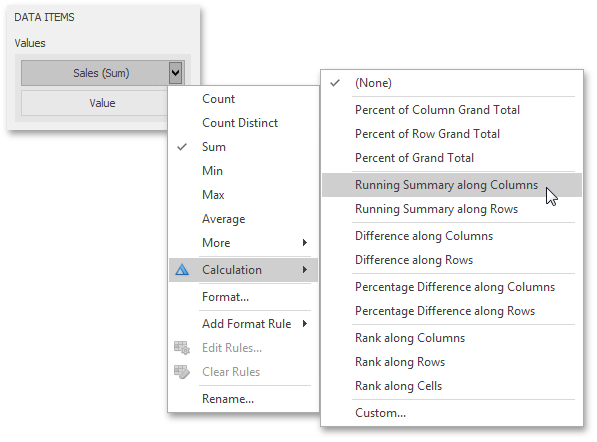The image above shows a calculation menu of the Pivot dashboard item. The following items are available.

• Percent of Column Grand Total - Calculates a contribution of individual measure values to a column grand total.
• Percent of Row Grand Total - Calculates a contribution of individual measure values to a row grand total.
• Percent of Grand Total - Calculates a contribution of individual measure values to a grand total.
• Running Summary along Columns - Calculates a cumulative total for measure values along columns (horizontally).
• Running Summary along Rows - Calculates a cumulative total for measure values along rows (vertically).
• Difference along Columns - Calculates differences between measure values along columns (horizontally).
• Difference along Rows - Calculates differences between measure values along rows (vertically).
• Percentage Difference along Columns - Calculates percent differences between measure values along columns (horizontally).
• Percentage Difference along Rows - Calculates percent differences between measure values along rows (vertically).
• Rank along Columns - Ranks measure values along columns (horizontally).
• Rank along Rows - Ranks measure values along rows (vertically).
• Rank along Cells - Ranks measure values along cells (throughout the entire pivot table).
• Custom... - Allows you to create a custom calculation by specifying various settings. Clicking this item invokes the Customize Calculation dialog that allows you to add additional customizations to calculations.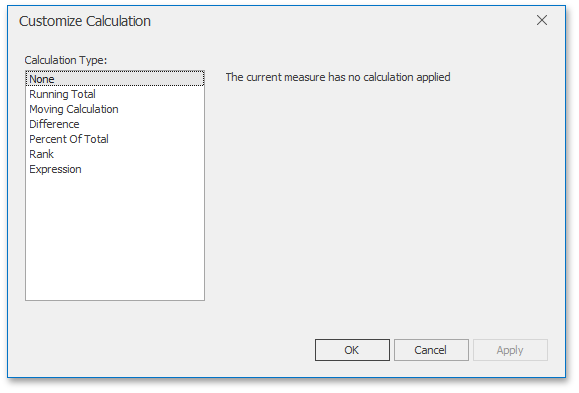##### NOTE

Note that the list of available items in this menu can be changed by the Dashboard Designer dynamically. For instance, if the Pivot dashboard item does not contain dimensions in the Rows section, menu items related to rows will be disabled.

## Running Total

The Running Total calculation can be used to compute a cumulative total for the specified measure across a window. For example, the Grid below displays cumulative sales across all quarters.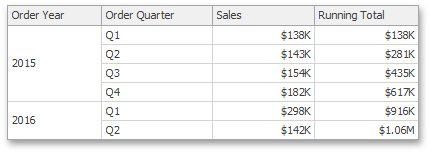The Customize Calculation dialog provides the following settings for the Running Total calculation.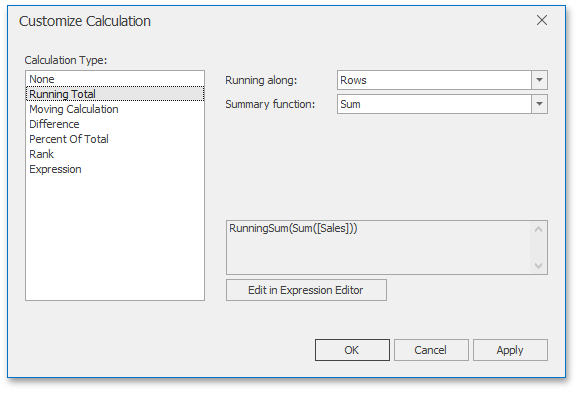• Running along - Specifies a window and direction used to calculate running totals.
• Summary function - Specifies a summary function used to apply calculation. To learn more about the available summary functions, see Summary Function Types in the summary function topic.

## Moving Calculation

The Moving calculation uses neighboring values to calculate a total. For example, the Grid below shows a moving average across all quarters.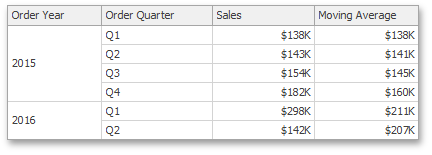The Customize Calculation dialog provides the following settings for the Moving calculation.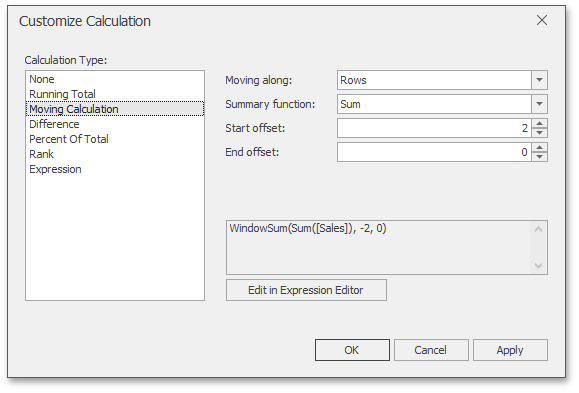• Moving along - Specifies a window and direction used to apply a calculation.
• Summary function - Specifies a summary function used to apply a calculation. To learn more about the available summary functions, see Summary Function Types in the summary function topic.
• Start offset / End offset - Specify start/end offsets from the currently processed value. For instance, if you specified offsets as 1/1, the previous and next values will be used along with the current value to apply the Moving calculation.

## Difference

The Difference calculation can be used to compute the difference between measure values across a window. For example, the Grid below shows absolute differences between quarterly sales.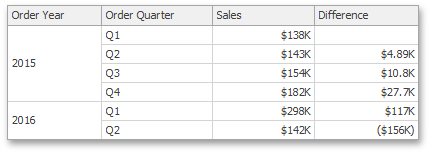The Customize Calculation dialog provides the following settings for the Difference calculation.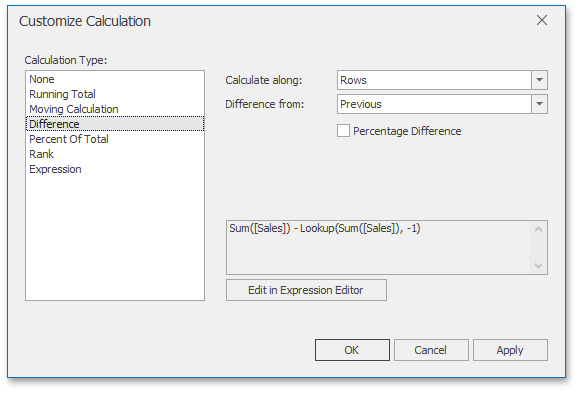• Calculate along - Specifies a window and direction used to calculate differences.
• Difference from - Specifies the value used to calculate the difference. The following values are available: Previous, Next, First and Last.

You can also use the Percentage Difference option to specify whether the absolute or percentage difference is displayed.

## Percent of Total

A calculation is used to compute a percentage of the total for the specified measure across a window. For example, the Grid below shows a contribution of individual quarterly sales to total sales.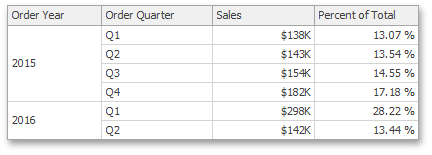The Customize Calculation dialog provides the following settings for the Percent of Total calculation.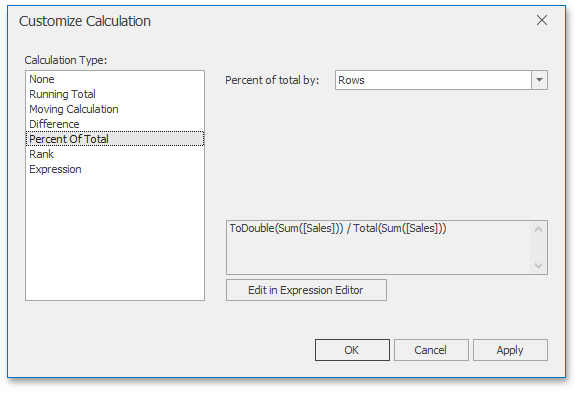## Rank

Use the Rank calculation to compute rankings for the specified measure across a window. For example, the Grid below shows a ranking of sales for individual quarters.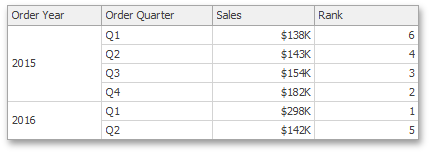The Customize Calculation dialog provides the following settings for the Rank calculation.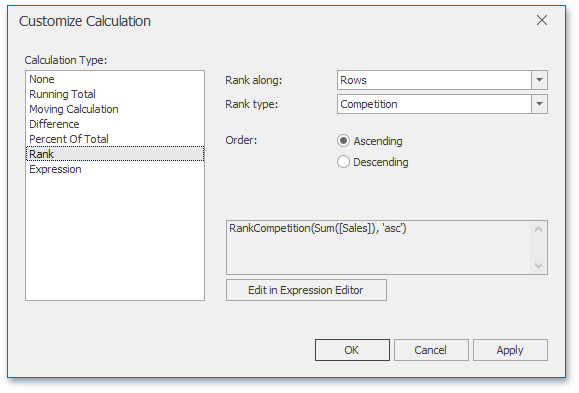• Rank along - Specifies a window and direction used to rank values.
• Rank type - Specifies the type of ranking. The following rank types are available: Unique, Competition, Dense, Modified and Percentile.
• Order - Specifies the order of ranking. You can select Ascending or Descending.

## Expression

Use Expression to specify a custom calculation by adding the required calculation functions inside the measure expression.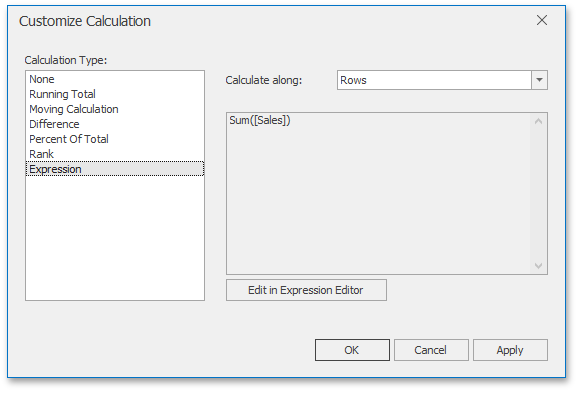Click the Edit in Expression Editor button to invoke the Expression Editor and specify the required expression.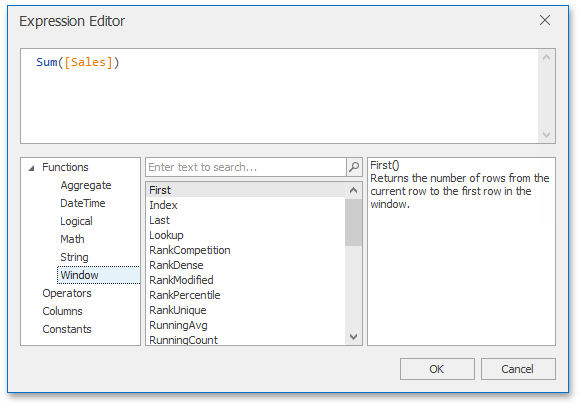The Expression type provides the Calculate along option that specifies a window and direction used to calculate differences. Note that this option is in effect if the expression contains a calculation function.

## Creating Calculations in Code

To apply a calculation to values of the required measure, perform the following steps.

The code snippet adds a new measure to the Pivot dashboard item and specifies a window calculation to compute the difference between measure values across a window. The calculation is performed along columns of the Pivot dashboard item.

``````using DevExpress.DashboardCommon;
using DevExpress.DashboardWin;
// ...
PivotDashboardItem pivotItem = dashboardViewer1.Dashboard.Items[pivotItemName] as PivotDashboardItem;
if (pivotItem != null)
{
Measure extendedPrice = new Measure("Extended Price")
{
Name = "Diff",
ShowGrandTotals = false
};
PivotWindowDefinition pivotWindowDefinition = new PivotWindowDefinition();
pivotWindowDefinition.DefinitionMode = PivotWindowDefinitionMode.Columns;
extendedPrice.WindowDefinition = pivotWindowDefinition;
extendedPrice.Calculation = new DifferenceCalculation() { DifferenceType = DifferenceType.Absolute };
}
``````

## API Members

API Description
Measure.Calculation Gets or sets a calculation applied to values of the current measure.
Measure.WindowDefinition Gets or sets the window definition used to apply a calculation to values of the current measure.
Measure.Expression Gets or sets the expression for the current measure.
Name Description
RunningTotalCalculation A running total calculation that is used to compute a cumulative total for the specified measure across a window.
MovingCalculation A moving calculation that is computed for the specified measure across a window.
DifferenceCalculation A difference calculation that is used to compute the difference between measure values across a window.
PercentOfTotalCalculation A calculation that is used to compute a percent of the total for the specified measure across a window.
RankCalculation A rank calculation that is used to compute rankings for the specified measure across a window.
MeasureCalculation Serves as the base class for classes that are measure calculations allowing you to apply specific computations to Measure values that are related to the currently processed value.
MeasureCalculationWindowDefinition Serves as the base class for classes that specify a window definition used to perform calculations within different dashboard items.